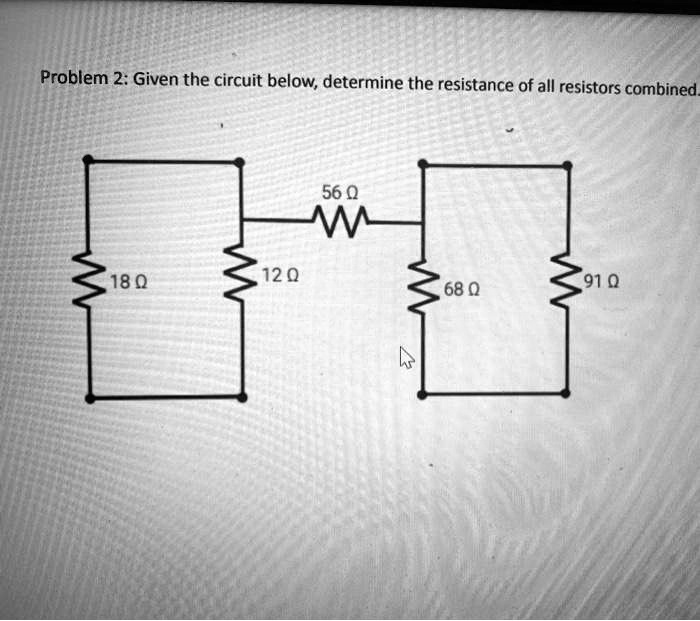5

# Problem 2: Given the circuit below, determine the resistance of all resistors combined_56 0...

## Question

###### Problem 2: Given the circuit below, determine the resistance of all resistors combined_56 0

Problem 2: Given the circuit below, determine the resistance of all resistors combined_ 56 0